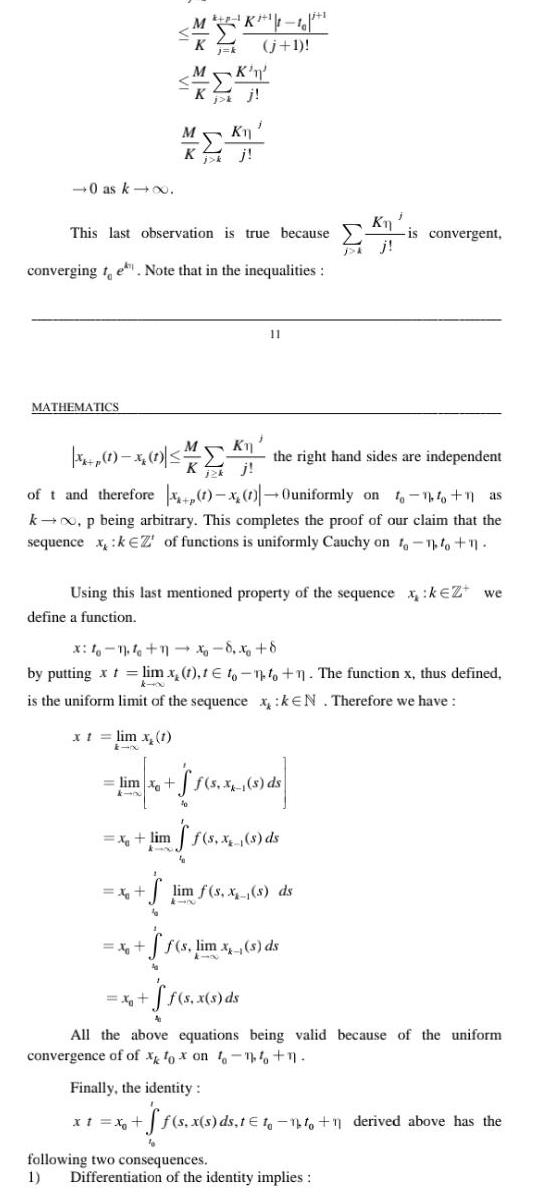Question:

# 0 as k MATHEMATICS This last observation is true because

Last updated: 9/18/20230 as k MATHEMATICS This last observation is true because converging te Note that in the inequalities M K 1 10 j 1 MK Kj XI K MK n Kj X 1 x 1 the right hand sides are independent Kj lim x X lim of t and therefore 1 x 1 Ouniformly on to 1 1 as k p being arbitrary This completes the proof of our claim that the sequence x kEZ of functions is uniformly Cauchy on to to 1 X Using this last mentioned property of the sequence xk Z we define a function x 4 1 1x8 x 8 by putting x t lim x 1 t to to 1 The function x thus defined is the uniform limit of the sequence x kEN Therefore we have lim x 1 x 11 x1 x f s X 5 5 1 de ff s x s ds ff s lim x s ds ff s x s ds Kn lim f s x s ds is convergent Finally the identity All the above equations being valid because of the uniform convergence of of x fox on to 1 1 s x s ds t 1 1 1 1 derived above has the following two consequences 1 Differentiation of the identity implies